Actions

(diff) ← Older revision | Latest revision (diff) | Newer revision → (diff)

of points

A quadruple of points on a straight line with cross ratio equal to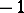. If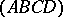is a harmonic quadruple of points, one says that the pair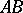harmonically divides the pair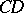, or that the pointsandare harmonically conjugate with respect to the pointsand; the pairsandare called harmonically conjugate.Figure: h046530a

A harmonic quadruple can be defined without recourse to metric concepts. Let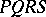be a quadrangle (see Fig.), letandbe the intersection points of the opposite sides, and letandbe the intersection points of the diagonals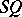and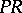ofwith the straight line. Then the quadruple of pointsis a harmonic quadruple. A quadruple of straight lines (or planes) passing through a single point (a single straight line) is called a harmonic quadruple of straight lines (planes) if a straight line intersects it in a harmonic quadruple of points.